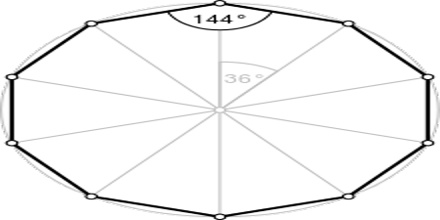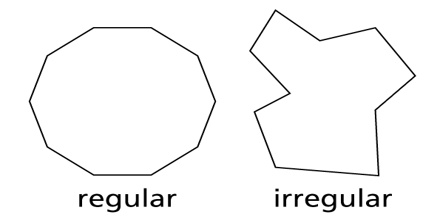A decagon is defined as a polygon with ten sides. The word “deca” itself means “ten”. A decagon has 10 sides and 10 internal angles. It has ten vertices as well. Decagons may be irregular or regular.Properties of Decagon

• Each angle in a regular decagon is equal to 144°.
• A regular decagon is one that has its 10 sides of equal length and 10 angles of equal measure.
• All sides are the same length (congruent) and all interior angles are the same size i.e congruent in case of regular decagon.
• Sum of all angles = 1440
• Number of triangles created by drawing the diagonals from a given vertex=8
• Straight sides, interior angle sum of 1440 degrees and exterior angle sum of 180 degrees, ten sides, flat, divides a plane into an inside and an outside.

Types

Regular Decagons

A regular decagon is referred as a decagon that has all 10 sides equal in length and has all 10 interior angles equal in measure. To find the measure of the central angle of a regular decagon, make a circle in the middle. A circle is 360 degrees around. Divide that by ten angles. 3600 / 10 = 360. So, the measure of the central angle of a regular decagon is 36 degrees.Irregular Decagons

Irregular decagons are those which have unequal sides and unequal angles. Few sides or angles may be same, but all of them are not.